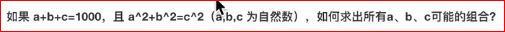2021-07-09 09:29

# Python:请问我这段代码哪里出现了问题？

``````a,b=0,0
while a<1000:
while b<1000:
c=1000-a-b
if a**2+b**2==c**2:
print(a,b,c)
b+=1
a+=1
``````• 写回答
• 好问题 提建议
• 追加酬金
• 关注问题
• 邀请回答

#### 2条回答默认 最新

•八云黧 2021-07-09 09:32
最佳回答

内层一直循环，直到b=1000时进入外层循环，因为不妈祖b<1000的控制条件，外层会直接跳过内层，让a不断自增到1000最终退出循环
得每次在外层循环将b的值重置为0

``````a,b=0,0
while a<1000:
b=0
while b<1000:
c=1000-a-b
if a**2+b**2==c**2:
print(a,b,c)
b+=1
a+=1

``````
评论
解决 1 无用
打赏 举报